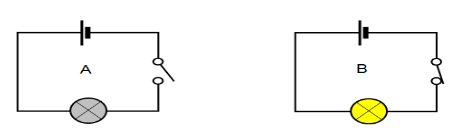Courses
Courses for Kids
Free study material
Offline Centres
MoreLast updated date: 08th Dec 2023
Total views: 281.7k
Views today: 4.81k

# When a switch is in $OFF$ position,$\left( i \right)$ Circuit starting from the positive terminal of the cell stops at the switch.$\left( {ii} \right)$ Circuit is open.$\left( {iii} \right)$ No current flows through it.$\left( {iv} \right)$ Current flows after some time.Choose the combination of correct answers from the following.A. all are correctB. $\left( {ii} \right)$ and$\left( {iii} \right)$ are correctC. only $\left( {iv} \right)$ is correctD. only $\left( i \right)$ and $\left( {ii} \right)$ are correctVerified
When the switch is in the $OFF$ position, the circuit is said to be open and when the circuit is open No current flows in the circuit. For example: consider a circuit consisting of a battery, a bulb and a switch as shown in the figure below,
In the first circuit the switch is in the $OFF$ position, the circuit is said to be open and the current will not flow and the bulb will be turned $OFF$. In the second circuit the switch in $ON$ position the circuit is said to be closed and the current will not flow and the bulb will be turned $ON$.